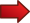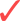# How to Factor

Learning how to factor is one of the most crucial skills you can learn. Factoring has so many applications, that you will be glad to take the time to learn all there is about it.

Factoring is normally something that we take for granted, and based upon different properties, such as the commutative, associative and distributive property. Those properties allow you to move around and group terms in a convenient way.

A quick refreshment about the commutative, associative and distributive property. For the real numbers $$x$$, $$y$$ and $$z$$, we have the following properties(Associativity of Addition) $$(x+y)+z = x+(y+z)$$Commutativity of Addition) $$x + y = y + x$$(Distributive Property) $$x \cdot (y+z) = x \cdot y + x \cdot z$$

where $$+$$ and $$\cdot$$ are the sum and product of real numbers, respectively.

## Why Is It Useful to Know How to Factor?

There are many reasons, but one of the crucial ones is that factoring gives us an easy way of solving equations. In fact, factoring is THE way we have to solve equations.

For example, consider the equation where we are trying to solve for $$x$$:

$\large xy +xz = 0$

How do we go about it? Well, we can use the distributive property to get:

$\large x y + x z = x(y+z) = 0$

Therefore, with this last expression $$x(y+z) = 0$$ we have an example of factoring. Indeed, we took the initial expression, $$xy+xz$$ and we factored it into $$x(y+z)$$.

So, now we need to solve an easier equation, which is $$x(y+z) = 0$$. Why is it easier? It is because now that we know that the product $$x(y+z)$$ is equal to zero, then one of the factors NEEDS to be equal to zero.

So, if we know that $$y+z = \not 0$$, then we know that we need to have that $$x = 0$$.LESSON : One advantage of factoring is being able to write an equation as a multiplication of factors that is equal to zero. Then, AT LEAST ONE OF THE FACTORS MUST BE ZERO.

For example, when we need to solve for $$x$$ in the following equation:

$\large 5x + 3x = 0$

we don't realize we are actually factoring when we do

$\large 5x + 3x = (5+3)x = 8x = 0$

so we have reduced our equation to a product of factors that is equal to zero: $$8x = 0$$. Since one of the factors $$8$$ is not equal to zero, then the only possible solution is $$x = 0$$.In other words : if you know how to factor, you will likely know how to solve equations .

## How to Factor Polynomials

The role of factoring should be clear by now, in terms of its usefulness to solve equations. The only problem is that there is not a generic, single strategy that can be used to factor ALL possible algebraic expressions.

So, normally, we will be happy to factor relatively simple expressions, but ideally, we would like to know how to factor as many expressions as we can.

The balance is reached with a very general class of expressions that we can, often times, factor very systematically. That class is the class of polynomials. For example, the expression

$\large 2x^2 + 5x + 3$

is a polynomial of degree 2. Or the expression below

$\large x^3 - 3x^2 + 4x+2$

is a polynomial of degree 3.

In general, a expression of the form

$\large a_n x^n + a_{n-1}x^{n-1} + ... + a_1 x + a_0$

is a polynomial of degree $$n$$. Naturally, the simpler the expression, the easier it will be to simplify, so we should try learn how to factor quadratic expressions first. This is, polynomials of degree two.

### EXAMPLE 1

$\large x^2 + x - 2$

This example will show you, on purpose, that it can be tricky to factor even the simplest expression, like the one above. What would you do to factor it?

What if I tell you, you need to add zero? It is kind ridiculous, right? Let's see:

$\large x^2 + x - 2 = x^2 + x + 0 - 2$

Do you agree with the above? I just added $$0$$. Nothing has changed. But, what if I tell you that $$0 = 2x - 2x$$? So then

$\large x^2 + x - 2 = x^2 + x + 0 - 2 = x^2 + x + (2x - 2x) - 2$

All the same! It works, because I added zero, so nothing changes. But now we expand it and group it:

$\large x^2 + x - 2 = x^2 + x + 0 - 2$ $\large = x^2 + x + (2x - 2x) - 2$ $\large = x^2 + x - 2x + 2x - 2$ $\large = x^2 + (x - 2x) + 2x - 2$ $\large = x^2 - x + 2x - 2$ $\large = x(x-1) + 2(x-1)$ $\large = (x+2)(x-1)$

So then, finally, $$x^2 + x - 2 = (x+2)(x-1)$$. Tricky? Perhaps, but that is one way to do it. Despite being a clever way to do it, we would prefer a more systematic way.

Clever tricks are nice, and all that, but usually we will prefer a systematic approach, that never fails. For quadratic polynomials (polynomials of degree 2), there is a systematic way of proceeding with the factoring:Step 1 : Given the quadratic expression $$ax^2 + bx + c$$, we first solve the equation

$\large ax^2 + bx + c = 0$Step 2 : If the solutions (roots) to the above equation are real (even if there is only one root), we call those roots $$x_1$$ and $$x_2$$. With these roots, we get the following factors:

$\large ax^2 + bx + c = a(x - x_1)(x - x_2)$

so the solutions $$x_1$$ and $$x_2$$ completely determine the factors.

Naturally in this case, as expected, solving a quadratic equation is tightly connected with factoring the quadratic equation.

### EXAMPLE 2

$\large x^2 - 4x + 3$

by computing its roots.

We start by solving the corresponding quadratic equation:

$\large x^2 - 4x + 3 = 0$

using the famous and well known quadratic formula :

$\large\displaystyle x = \frac{-b \pm \sqrt{b^2 - 4ac}}{2a}$ $\large\displaystyle = \frac{-(-4) \pm \sqrt{(-4)^2 - 4(1)(3)}}{2(1)}$ $\large\displaystyle = \frac{4 \pm \sqrt{16 - 12}}{2}$ $\large\displaystyle = \frac{4 \pm \sqrt{4}}{2}$ $\large\displaystyle = \frac{4 \pm 2}{2}$

which implies that the solutions (roots) are $$x_1 = 1$$ and $$x_2 = 1$$. Then, the quadratic expression $$x^2 - 4x + 3$$ can be factored as follows:

$\large x^2 - 4x + 3 = a(x - x_1)(x - x_2) = (x-1)(x-3)$

Observe that in this case the term multiplying the $$x^2$$ term is 1, so then in this case $$a = 1$$.

## Factoring Polynomials with degree greater than 2

So, to factor quadratic polynomials I just compute the roots of the corresponding quadratic equation. How do I factor polynomials of higher degree?? Using exactly the same method .Step 1: Given the polynomial expression $$a_n x^n + a_{n-1}x^{n-1} + ... + a_1 x + a_0$$, we first solve the equation

$\large a_n x^n + a_{n-1}x^{n-1} + ... + a_1 x + a_0 = 0$Step 2: If the solutions (roots) to the above equation are real (even if they are repeated), we call those roots $$x_1$$, $$x_2$$, ..., $$x_n$$. With these roots, we get the following factors:

$\large a_n x^n + a_{n-1}x^{n-1} + ... + a_1 x + a_0 = a_n(x - x_1)(x - x_2)\cdots (x - x_n)$

So, it would seem that it is equally simple to factor a polynomial of degree 2 than it is to factor a polynomial of degree 10. Theoretically, the answer is yes.

The only problem is that there is not a simple, close algebraic formula that can solve roots for a polynomial equation of degree 5 or higher.

Sometimes, we can solve higher degree equations by looking at the graph, or even using the calculator. For example, check the graph below:Graphically, we can see that the polynomial crosses the x-axis at three points: $$x_1 = -1$$, $$x_2 = 1$$ and $$x_3 = 3$$, so these are the roots.

So then, we know that the polynomial must be of the form $$p(x) = a(x+1)(x-1)(x-3)$$. We would need to know one more point to know the constant $$a$$.

We are just scratching the surface with the concept of factoring, although there is not much more than can be done for general expressions. The best we can do is to give a systematic approach to factor polynomials.

But, having a general treatment to factor polynomials is not a minor thing, and the idea of using the roots to factor a polynomial is nothing less than the Fundamental Theorem of Algebra. So, at least by the title, you can tell it is not little.

### Factoring General Expressions

There are no general rules to factor general expressions. We need to play by ear and try to exploit the structure of the expression. Sometimes we can factor, sometimes we cannot. It all depends on the expression. The only general rule is to try to group and try to find common factors so to further group and simplify.

### How to Factor by Grouping

That is the first example we did. Say you have:

$\large x^2 - x + 2x - 2$

so we group the first two terms and the last two terms to get:

$\large (x^2 - x) + (2x - 2)$

and each of these groups can be factored as

$\large x(x - 1) + 2(x - 1)$

and now we have two terms that have a common factor $$x-1$$, so we factor it as

$\large (x+2)(x - 1)$

Sometimes it is more practical to use a calculator to find the factors. You can use our quadratic equation solver to find the factors of a quadratic expression.

Notice that there are several techniques that can help you when you need to factor a expression, depending of its structure. One of those is the method of factor by grouping which, when it works, can simplify the simplification process a whole lot.